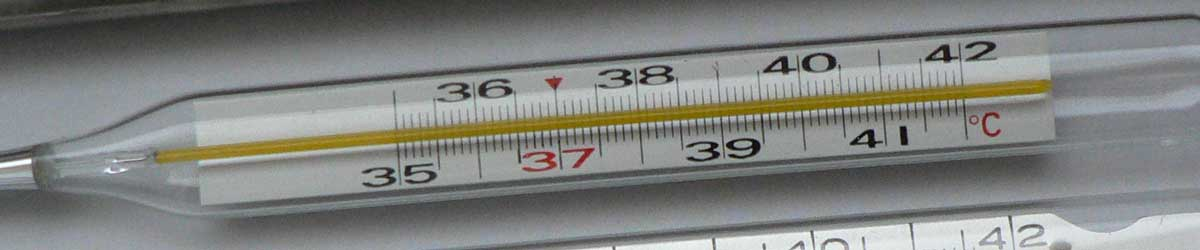# Celsius to Newton Conversion

Enter the temperature in Celsius below to get the value converted to Newton.

Results in Degrees Newton:20 °C = 6.6 °N
Do you want to convert Newton to Celsius?

## How to Convert Celsius to NewtonConvert degrees Celsius to degrees Newton with this simple formula:

degrees Newton = [°C] × 0.33000

Insert the [°C] temperature measurement in the formula and then solve to find the result.

For example, let's convert 50 °C to degrees Newton:

50 °C = ( 50 × 0.33000 ) = 16.5 °N

## Celsius

The Celsius temperature scale, also commonly referred to as the centigrade scale, is defined in relation to the kelvin. Specifically, degrees Celsius is equal to kelvins minus 273.15.

The degree Celsius is the SI derived unit for temperature in the metric system. A degree Celsius is sometimes also referred to as a degree centigrade. Celsius can be abbreviated as C; for example, 1 degree Celsius can be written as 1 °C.

### Important Temperatures In Degrees Celsius

TemperatureDegrees Celsius
Absolute Zero-273.15 °C
Freezing Point of Water0 °C
Triple Point of Water0.01 °C
Boiling Point of Water100 °C

## Newton

The Newton scale is a temperature scale that sets a fixed zero point equal to the freezing point water. The scale uses both arithmetic progression and geometric progression. The scale defines 18 reference points and the corresponding "degrees of heat" and geometric progressions.

Newton can be abbreviated as N; for example, 1 degree Newton can be written as 1 °N.

## Celsius to Newton Conversion Table

Celsius measurements converted to Newton
Celsius Newton
-50 °C -16.5 °N
-40 °C -13.2 °N
-30 °C -9.9 °N
-20 °C -6.6 °N
-10 °C -3.3 °N
0 °C 0 °N
10 °C 3.3 °N
20 °C 6.6 °N
30 °C 9.9 °N
40 °C 13.2 °N
50 °C 16.5 °N
60 °C 19.8 °N
70 °C 23.1 °N
80 °C 26.4 °N
90 °C 29.7 °N
100 °C 33 °N
110 °C 36.3 °N
120 °C 39.6 °N
130 °C 42.9 °N
140 °C 46.2 °N
150 °C 49.5 °N
160 °C 52.8 °N
170 °C 56.1 °N
180 °C 59.4 °N
190 °C 62.7 °N
200 °C 66 °N
300 °C 99 °N
400 °C 132 °N
500 °C 165 °N
600 °C 198 °N
700 °C 231 °N
800 °C 264 °N
900 °C 297 °N
1,000 °C 330 °N

## References

1. National Institute of Standards and Technology, SI Units - Temperature, https://www.nist.gov/pml/weights-and-measures/si-units-temperature